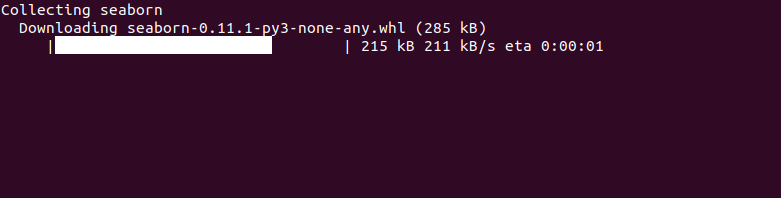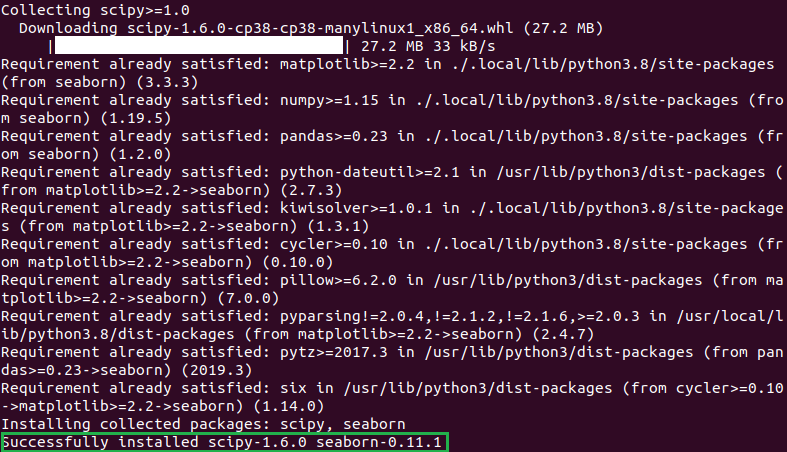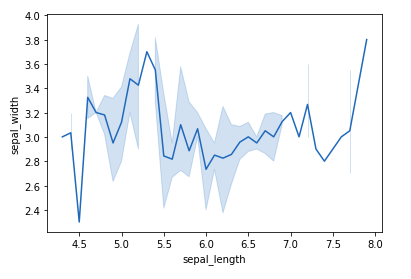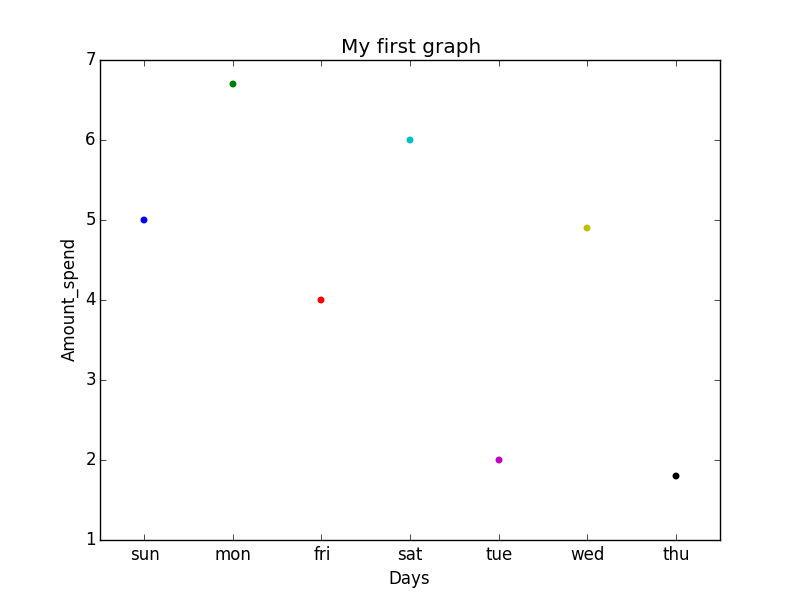Open in App
Not now

# How to Install Seaborn on Linux?

• Last Updated : 19 Nov, 2021

Seaborn is a library mostly used for statistical plotting in Python. It is built on top of Matplotlib and provides beautiful default styles and color palettes to make statistical plots more attractive.

### Seaborn Dependencies:

Seaborn has the following dependencies:

### Installing Seaborn on Linux:

Use the below command in the terminal to install Seaborn:

`pip install seaborn`

In the terminal, it will look like this:After the installation is completed you will get a successfully installed message at the end of the terminal as shown below:After the installation let us see an example of a simple plot using Seaborn. We will be plotting a simple line plot using the iris dataset. Iris dataset contains five columns such as Petal Length, Petal Width, Sepal Length, Sepal Width, and Species Type. Iris is a flowering plant, the researchers have measured various features of the different iris flowers and recorded them digitally.

Example:

## Python3

 `# importing packages``import` `seaborn as sns` `# loading dataset``data ``=` `sns.load_dataset(``"iris"``)` `# draw lineplot``sns.lineplot(x``=``"sepal_length"``, y``=``"sepal_width"``, data``=``data)`

Output:In the above example, a simple line plot is created using the lineplot() method.

Example 2:  Plotting categorical scatter plots with Seaborn using Matplotlib.

## Python3

 `# Python program to illustrate``# Plotting categorical scatter``# plots with Seaborn` `# importing the required module``import` `matplotlib.pyplot as plt``import` `seaborn as sns` `# x axis values``x ``=``[``'sun'``, ``'mon'``, ``'fri'``, ``'sat'``, ``'tue'``, ``'wed'``, ``'thu'``]` `# y axis values``y ``=``[``5``, ``6.7``, ``4``, ``6``, ``2``, ``4.9``, ``1.8``]` `# plotting strip plot with seaborn``ax ``=` `sns.stripplot(x, y);` `# giving labels to x-axis and y-axis``ax.``set``(xlabel ``=``'Days'``, ylabel ``=``'Amount_spend'``)` `# giving title to the plot``plt.title(``'My first graph'``);` `# function to show plot``plt.show()`

Output:Here,

• Categorical data is represented on the x-axis and values correspond to them represented through the y-axis.
• .striplot() function is used to define the type of the plot and to plot them on canvas using.
• .set() function is used to set labels of x-axis and y-axis.
• .title() function is used to give a title to the graph.
• To view plot we use .show() function.

My Personal Notes arrow_drop_up